# Selina Solutions Concise Maths Class 10 Chapter 7 Ratio and Proportion Exercise 7(C)

Some important properties of proportion like invertendo, alternendo, componendo, dividendo and other direct applications are the concepts discussed in this exercise. The exercise problems pertain to these topics and are very important for exams. To get complete knowledge about these concepts and attain a strong grip over other chapters, the Selina Solutions for Class 10 Maths is the right tool for it. Further, the solutions to this exercise are available in the Concise Selina Solutions for Class 10 Maths Chapter 7 Ratio and Proportion Exercise 7(C) PDF in the links attached below.

## Selina Solutions Concise Maths Class 10 Chapter 7 Ratio and Proportion Exercise 7(C) Download PDF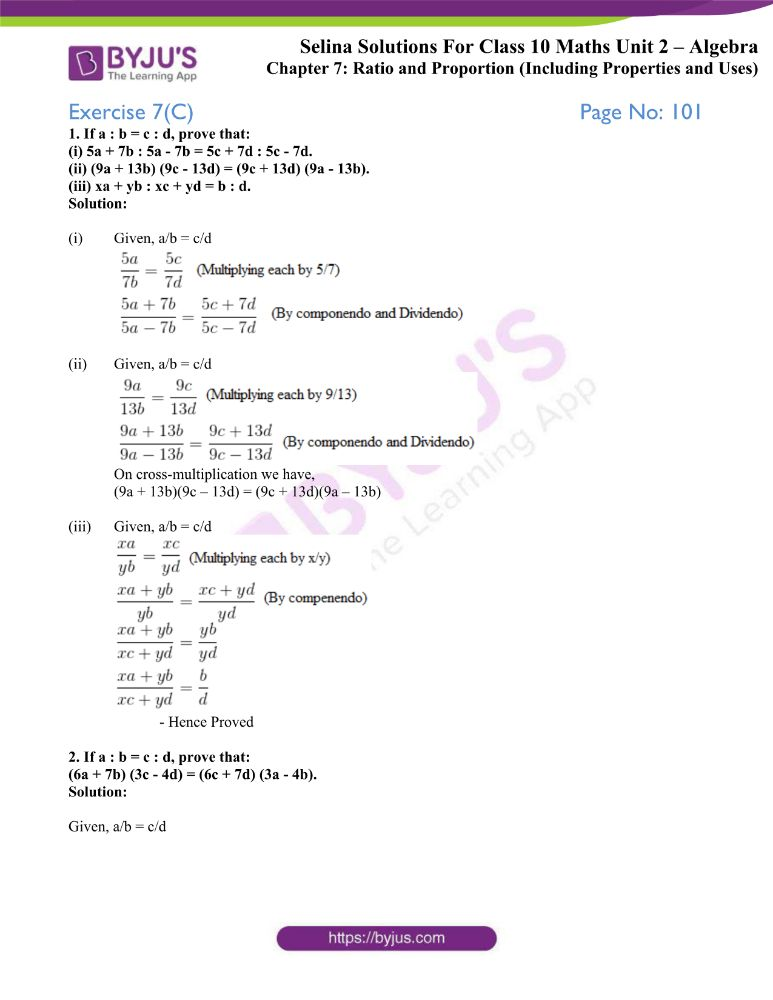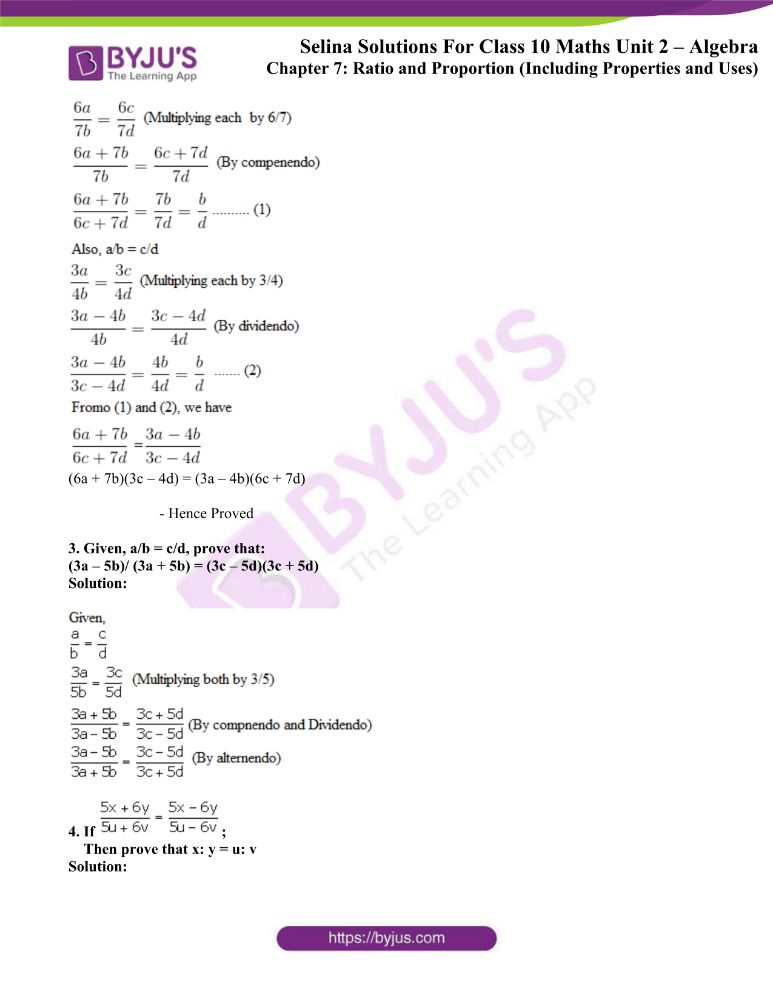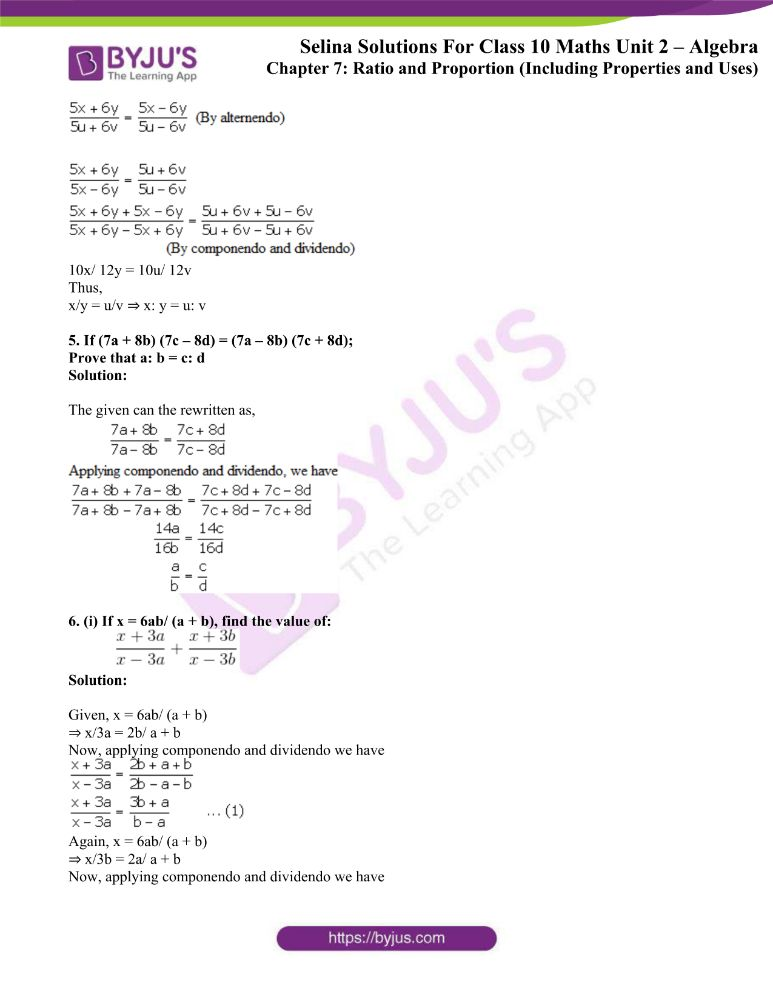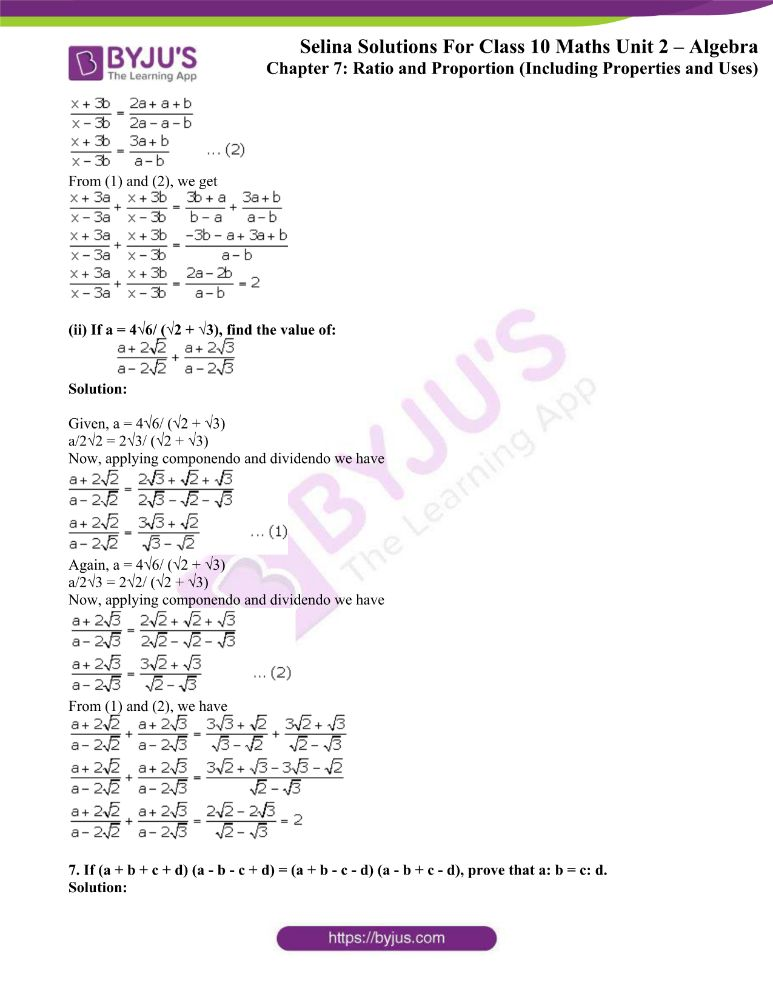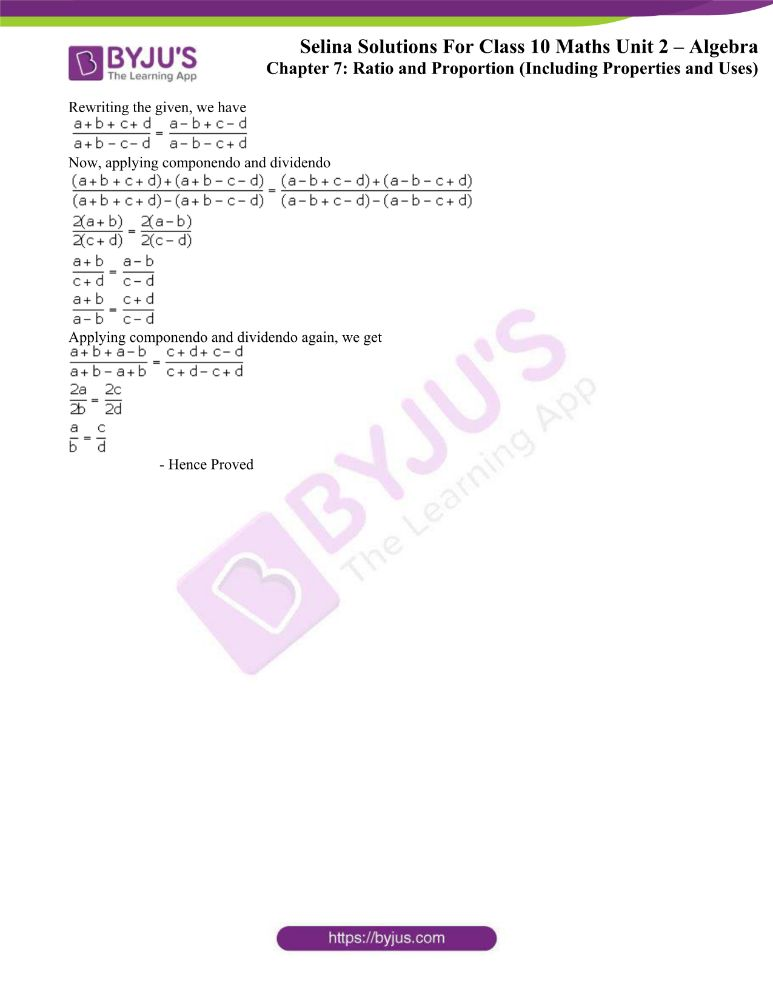### Access other exercises of Selina Solutions Concise Maths Class 10 Chapter 7 Ratio and Proportion

Exercise 7(A) Solutions

Exercise 7(B) Solutions

Exercise 7(D) Solutions

### Access Selina Solutions Concise Maths Class 10 Chapter 7 Ratio and Proportion Exercise 7(C)

1. If a : b = c : d, prove that:

(i) 5a + 7b : 5a – 7b = 5c + 7d : 5c – 7d.

(ii) (9a + 13b) (9c – 13d) = (9c + 13d) (9a – 13b).

(iii) xa + yb : xc + yd = b : d.

Solution:

(i) Given, a/b = c/d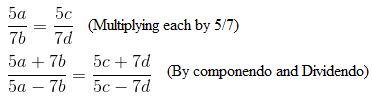(ii) Given, a/b = c/dOn cross-multiplication we have,

(9a + 13b)(9c – 13d) = (9c + 13d)(9a – 13b)

(iii) Given, a/b = c/d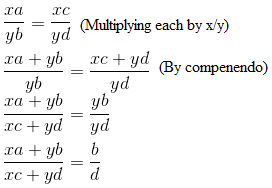– Hence Proved

2. If a : b = c : d, prove that:

(6a + 7b) (3c – 4d) = (6c + 7d) (3a – 4b).

Solution:

Given, a/b = c/d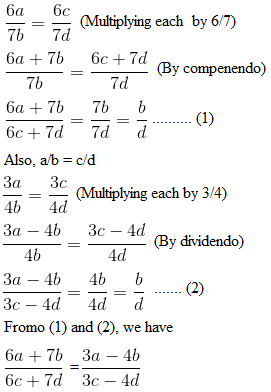(6a + 7b)(3c – 4d) = (3a – 4b)(6c + 7d)

– Hence Proved

3. Given, a/b = c/d, prove that:

(3a – 5b)/ (3a + 5b) = (3c – 5d)(3c + 5d)

Solution: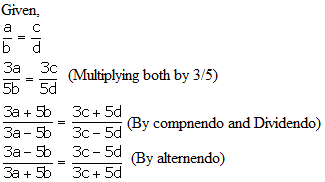4. If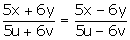;

Then prove that x: y = u: v

Solution: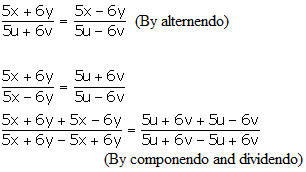10x/ 12y = 10u/ 12v

Thus,

x/y = u/v ⇒ x: y = u: v

5. If (7a + 8b) (7c – 8d) = (7a – 8b) (7c + 8d);

Prove that a: b = c: d

Solution:

The given can the rewritten as,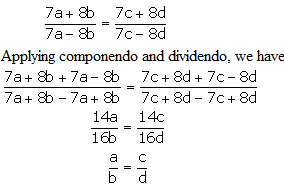6. (i) If x = 6ab/ (a + b), find the value of: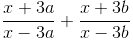Solution:

Given, x = 6ab/ (a + b)

⇒ x/3a = 2b/ a + b

Now, applying componendo and dividendo we have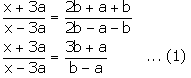Again, x = 6ab/ (a + b)

⇒ x/3b = 2a/ a + b

Now, applying componendo and dividendo we have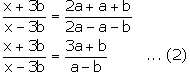From (1) and (2), we get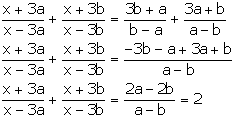(ii) If a = 4√6/ (√2 + √3), find the value of: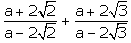Solution:

Given, a = 4√6/ (√2 + √3)

a/2√2 = 2√3/ (√2 + √3)

Now, applying componendo and dividendo we have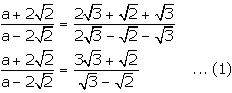Again, a = 4√6/ (√2 + √3)

a/2√3 = 2√2/ (√2 + √3)

Now, applying componendo and dividendo we have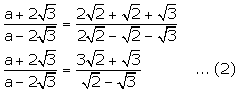From (1) and (2), we have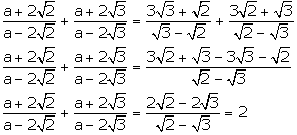7. If (a + b + c + d) (a – b – c + d) = (a + b – c – d) (a – b + c – d), prove that a: b = c: d.

Solution:

Rewriting the given, we have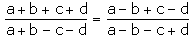Now, applying componendo and dividendo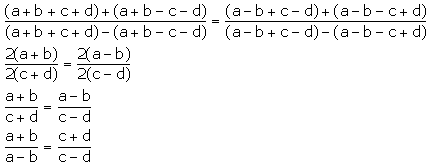Applying componendo and dividendo again, we get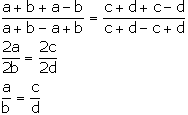– Hence Proved